### COV Statement

COV assignment <, assignment …> ;

where assignment represents var-list < *var-list2 > < = parameter-spec>

The COV statement is a subsidiary model specification statement for the confirmatory FACTOR and LINEQS models. In the LINEQS model, the COV statement defines the covariances among the exogenous variables, including errors and disturbances. In the confirmatory FACTOR model, the COV statement defines the factor covariances. In each assignment of the COV statement, you specify variables in the var-list and the var-list2 lists, followed by the covariance parameter specification in the parameter-spec list. The latter two specifications are optional.

You can specify the following five types of the parameters for the covariances:

• an unnamed free parameter

• an initial value

• a fixed value

• a free parameter with a name provided

• a free parameter with a name and initial value provided

Consider a LINEQS model with exogenous variables V1, V2, V3, and V4. The following COV statement shows the five types of specifications in five assignments:

cov V2 V1 ,
V3 V1 = (0.3),
V3 V2 = 1.0,
V4 V1 = phi1,
V4 V2 = phi2(0.2);


In this statement, cov(V2,V1) is specified as an unnamed free parameter. For this covariance, PROC CALIS generates a parameter name with the _Parm prefix and appended with a unique integer (for example, _Parm1). cov(V3,V1) is an unnamed free parameter but with an initial value of 0.3. PROC CALIS also generates a parameter name for this covariance. cov(V3,V2) is a fixed value of 1.0. This value stays the same in the estimation. cov(V4,V1) is a free parameter named phi1. cov(V4,V2) is a free parameter named phi2 with an initial value of 0.2.

Note that the var-list and var-list2 lists on the left-hand side of the equal sign of the COV statement should contain only names of exogenous variables. Hence, the COV statement is different from the PCOV statement in which you can list both exogenous and endogenous variables, although the COV and PCOV statements share the same syntax.

You can use the COV statement for specifying covariance parameters in the FACTOR and LINEQS models. In the FACTOR model, the COV statement specifies the covariances among latent factors. In the LINEQS model, the COV statement specifies the covariances among all observed or latent exogenous variables, including error and disturbance terms.

If you specify only the var-list list, then you are specifying the so-called within-list covariances. If you specify both of the var-list and var-list2 lists, then you are specifying the so-called between-list covariances. An asterisk is used to separate the two variable lists. You can use one of these two alternatives to specify the covariance parameters. Figure 27.2 illustrates the within-list and between-list covariance specifications.

Figure 27.2: Within-List and Between-List Covariances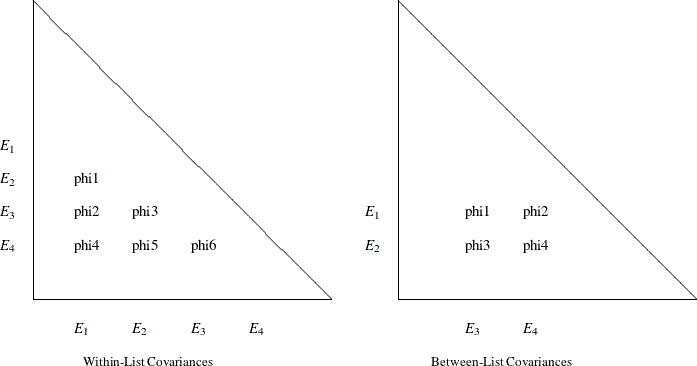#### Within-List Covariances

The left panel of the figure shows that the same set of four variables are used in both the rows and columns. This yields six nonredundant covariances to specify. In general, with a var-list list with k variables in the COV statement, there are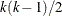distinct covariance parameters you can specify. The variable order of the var-list list is important. For example, the left panel of Figure 27.2 corresponds to the following COV statement specification:

cov E1-E4 = phi1-phi6;


This specification is equivalent to the following specification:

cov E2 E1 = phi1,
E3 E1 = phi2, E3 E2 = phi3,
E4 E1 = phi4, E4 E2 = phi5, E4 E3 = phi6;


Another way to assign distinct parameter names with the same prefix is to use the so-called prefix-name. For example, the following COV statement specification is exactly the same as the preceding specification:

cov E1-E4 = 6*phi__; /* phi with two trailing underscores */


In the COV statement, phi_ _ is a prefix-name with the root phi. The notation 6* means this prefix-name is applied six times, resulting in a generation of the six parameter names phi1, phi2, …, phi6 for the six covariance parameters.

The root of the prefix-name should have few characters so that the generated parameter name is not longer than 32 characters. To avoid unintentional equality constraints, the prefix-names should not coincide with other parameter names.

You can also specify the within-list covariances as unnamed free parameters, as shown in the following statement:

cov E1-E4;


This specification is equivalent to the following specification:

cov E2 E1,
E3 E1, E3 E2,
E4 E1, E4 E2, E4 E3;


#### Between-List Covariances

The right panel of Figure 27.2 illustrates the application of the between-list covariance specification. The set of row variables is different from the set of column variables. You intend to specify the cross covariances of the two sets of variables. There are four of these covariances in the figure. In general, with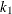and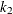variable names in the two variable lists (separated by an asterisk) in a COV statement, there are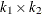distinct covariances to specify. Again, variable order is very important. For example, the right panel of Figure 27.2 corresponds to the following between-list covariance specification:

cov E1 E2 * E3 E4 = phi1-phi4;


This is equivalent to the following specification:

cov  E1 E3 = phi1, E1 E4 = phi2,
E2 E3 = phi3, E2 E4 = phi4;


You can also use the prefix-name specification for the same specification, as shown in the following statement:

cov  E1 E2 * E3 E4 = 4*phi__ ; /* phi with two trailing underscores */


#### Mixed Parameter Lists

You can specify different types of parameters for the list of covariances. For example, you use a list of parameters with mixed types in the following statement:

cov E1-E4 = phi1(0.1)  0.2  phi3  phi4(0.4) (0.5) phi6;


This specification is equivalent to the following specification:

cov E2 E1 = phi1(0.1) ,
E3 E1 = 0.2       , E3 E2 = phi3,
E4 E1 = phi4(0.4) , E4 E2 = (0.5), E4 E3 = phi6;


Notice that an initial value that follows a parameter name is associated with the free parameter. Therefore, in the original mixed list specification, 0.1 is interpreted as the initial value for the parameter phi1, but not as the initial estimate for the covariance between E3 and E1. Similarly, 0.4 is the initial value for the parameter phi4, but not the initial estimate for the covariance between E4 and E2.

However, if you indeed want to specify that phi1 is a free parameter without an initial value and 0.1 is an initial estimate for the covariance between E3 and E1 (while keeping all other things the same), you can use a null initial value specification for the parameter phi1, as shown in the following statement:

cov E1-E4 = phi1() (0.1)  phi3  phi4(0.4) (0.5) phi6;


This way 0.1 becomes the initial estimate for the covariance between E3 and E1. Because a parameter list with mixed types might be confusing, you can break down the specifications into separate assignments to remove ambiguities. For example, you can use the following equivalent specification:

cov E2 E1 = phi1 ,
E3 E1 = (0.1)     , E3 E2 = phi3,
E4 E1 = phi4(0.4) , E4 E2 = (0.5), E4 E3 = phi6;


#### Shorter and Longer Parameter Lists

If you provide fewer parameters than the number of covariances in the variable lists, all the remaining parameters are treated as unnamed free parameters. For example, the following specification assigns a fixed value to cov(E1,E3) while treating all the other three covariances as unnamed free parameters:

cov  E1 E2 * E3 E4 = 1.0;


This specification is equivalent to the following specification:

cov  E1 E3 = 1.0, E1 E4, E2 E3, E2 E4;


If you intend to fill up all values by the last parameter specification in the list, you can use the continuation syntax [...], [..], or [.], as shown in the following example:

cov  E1 E2 * E3 E4 = 1.0 phi [...];


This means that cov(E1,E3) is a fixed value of 1 and all the remaining three covariances are free parameter named phi. The last three covariances are thus constrained to be equal by using the same parameter name.

However, you must be careful not to provide too many parameters. For example, the following specification results in an error:

cov  E1 E2 * E3 E4 = 1.0 phi2(2.0) phi3 phi4 phi5 phi6;


The parameters after phi4 are excessive.

#### Default Covariance Parameters

In the confirmatory FACTOR model, by default all factor covariances are free parameters. In the LINEQS model, by default all covariances among exogenous manifest and latent variables (excluding error or disturbance variables) are also free parameters. For these default free parameters, PROC CALIS generate the parameter names with the _Add prefix and appended with unique integer suffixes. You can also use the COV statement specification to override these default covariance parameters in situations where you want to set parameter constraints, provide initial or fixed values, or make parameter references.

Another type of default covariances are fixed zeros. In the LINEQS model, covariances among errors or disturbances are all fixed zeros by default. Again, you can override the default fixed values by providing explicit specification of these covariances in the COV statement.

#### Modifying a Covariance Parameter Specification from a Reference Model

If you define a new model by using a reference (old) model in the REFMODEL statement, you might want to modify some parameter specifications from the COV statement of the reference model before transferring the specifications to the new model. To change a particular covariance specification from the reference model, you can simply respecify the same covariance with the desired parameter specification in the COV statement of the new model. To delete a particular covariance parameter from the reference model, you can specify the desired covariance with a missing value specification in the COV statement of the new model.

For example, suppose that the covariance between variables V1 and V2 is specified in the reference model but you do not want this covariance specification be transferred to the new model. You can use the following COV statement specification in the new model:

cov  V1 V2 = .;


Note that the missing value syntax is valid only when you use it with the REFMODEL statement. See the section Modifying a LINEQS Model from a Reference Model for a more detailed example of the LINEQS model respecification with the REFMODEL statement. See the section Modifying a FACTOR Model from a Reference Model for a more detailed example of the FACTOR model respecification with the REFMODEL statement.

As discussed in a preceding section, PROC CALIS generates some default free covariance parameters for the LINEQS and FACTOR models if you do not specify them explicitly in the COV statement. When you use the REFMODEL statement for defining a reference model, these default free covariance parameters in the old (reference) model are not transferred to the new model. Instead, the new model generates its own set of default free covariance parameters after it is resolved from the reference model, the REFMODEL statement options, the RENAMEPARM statement, and the COV statement specifications in the new model. This also implies that if you want any of the covariance parameters to be constrained across the models by means of the REFMODEL specification, you must specify them explicitly in the COV statement of the reference model so that the same covariance specification is transferred to the new model.